# Motorbike circuit

On a 2,550 m long circuit, two motorcycles go at such speeds that they meet every minute if they go against each other and run every 5 minutes if they go in the same direction. Find their speeds.

v1 =  91.8 km/h
v2 =  61.2 km/h

### Step-by-step explanation: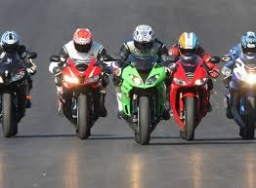Did you find an error or inaccuracy? Feel free to write us. Thank you!Tips to related online calculators
Do you have a linear equation or system of equations and looking for its solution? Or do you have a quadratic equation?
Do you want to convert velocity (speed) units?
Do you want to convert time units like minutes to seconds?

## Related math problems and questions:

• Two carsTwo cars (trucks and passenger) drove simultaneously from two places 50 km away. If they go against each other, they will meet in 30 minutes. If they follow each other in the same direction, the car will catch up with the truck in 2.5 hours. What is the s
• Two trains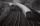There are two trains running the same distance. 1st train will travel it in 7 hours 21minutes. 2nd the train will travel 5 hours 57minutes and 34 seconds and it is 14 km/h faster than the first train. What are speeds of trains and how long is this railway
• Two trainsThrough the bridge, long l = 240m, the train passes through the constant speed at time t1 = 21s. A train running along the traffic lights at the edge of the bridge passes the same speed at t2 = 9s. a) What speed v did the train go? b) How long did it take
• Double-track line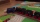A 160 m long passenger train runs on a double-track line in one direction at a constant speed of 54 km/h, and a 240 m long express train in the opposite direction. a) How fast is the express train if passing the passenger train driver for 6 s? b) How long
• Car and motorcyclistA car and a motorcyclist rode against each other from a distance of 190 km. The car drove 10km/h higher than the motorcyclist and started half an hour later. It met a motorcyclist in an hour and thirty minutes. Determine their speeds.
• Long hot dog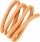In Kostelec above Black Forest, they made a record-long hot dog. When slowly moved it to the square, one of the spectators decided to measure its length: the size of his footprint is 28 cm; he measured 20 feet in travel direction, counted 5 feet against t
• Cars speedsDistance from city A to city B is 108 km. Two cars were simultaneously started from both places. The speed of a car coming from city A was 2 km/h higher than the second car. What was the speed of each car if they met in 54 minutes?
• The escalator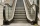I run up the escalator at a constant speed in the stairs' direction and write down the number of steps A we climbed. Then we turn around and run it at the same constant speed in the opposite direction and write down the number of steps B that I climbed. I
• Car loop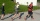The toy car runs at an average speed of 2 m/s. In a minute, it will pass the entire circuit five times. How long is the loop?
• Aircrafts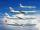Above the town hall tower flew the plane with constant speed 510 km/h and 15 minutes later the second plane at speed of 703 km/h. How long and how far from the town hall will be aircrafts caught up?
• The train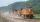The train passed the observer in the 20s and passed through a 1500m tunnel in 2 minutes. Calculate the speed (km/h) and train length.
• Peleton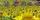The cycling race was run at an average speed of 45 km/h. One cyclist lost with defect 8 minutes. How long and how far he must go at speed 51 km/h to catch the peloton again?
• Two trains meetFrom A started at 7:15 express train at speed 85 km/h to B. From B started passenger train at 8:30 in the direction to A and at speed 55 km/h. The distance A and B are 386 1/4 km. At what time and at what distance from B the two trains meet?
• Two planes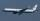Two planes are flying from airports A and B, 420 km distant from each other. The plane from A took off 15 minutes later and flies at an average speed of 40 km/h higher than the plane from B. Determine the average speed of these two aircraft if you know th
• Cars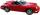Cars started at 7:00 AM. From point A car run at 40 km per hour, from B car run 70 km per hour. Between A and B is a 250 km long road. When and where will meet (measured from point A)?
• Paul and Honza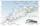Honza went to chat on a bike without a break 4.0 hours. Paul the same way without breaks 50 minutes longer. The difference between the speeds of the two boys was the one kilometer per hour. How fast was Paul, if you know that the speed of the boys did not
• Long bridge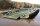Roman walked on the bridge. When he heard the whistle, he turned and saw running Kamil at the beginning of the bridge. If he went to him, they would meet in the middle of the bridge. Roman, however, rushed and so did not want to waste time returning 150m.